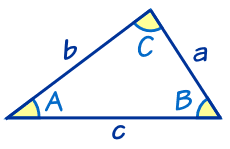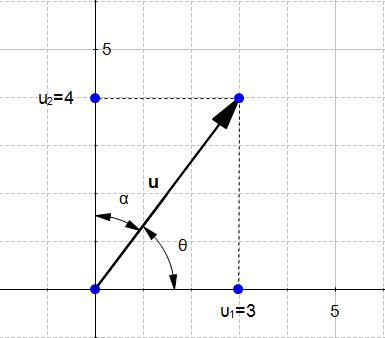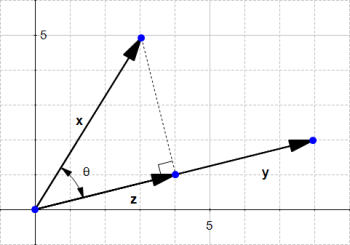## A bit of Trigonometry

### Right angle triangle• sin(θ) = Opposite / Hypotenuse
• cos(θ) = Adjacent / Hypotenuse
• tan(θ) = Opposite / Adjacent
• Pythagoras Theorem: $$a^2 + b^2 = c^2$$

### Other triangle• The Law of Cosines: $$c^2=a^2+b^2$$
• The Law of Sines: $$\frac{a}{sinA}=\frac{b}{sinB}=\frac{c}{sinC}$$

## Vector Magnitude and Direction• Magnitude of u (distance): $$\|\vec{u}\|=\sqrt{\|u_1\|^2+\|u_2\|^2}$$ (ie. Pythagorean Theorem)
• Direction vector of u(u1, u2) is a unit vector with the same direction: $$\vec{w}=(\frac{u1}{\|u\|},\frac{u2}{\|u\|})$$
• For right triangle, you also have the following properties: $$cos(\theta)=\frac{u_1}{\|u\|}; cos(\alpha)=\frac{u_2}{\|u\|}$$

## Dot product of a and b = scalar• Dot product of x and y:
• $$\vec{x}\cdot\vec{y}=\|x\|\|y\|cos\theta=x_1y_1+x_2y_2$$
• It involves multiplying two vectors together to get a scalar, not another vector (for this reason, the dot product is often referred to as a scalar product).
• Two vectors a and b are orthogonal if and only if $$\vec{a}\cdot\vec{b} = 0$$

## Cross product of a and b = another vector• it multiplies two vectors together to obtain a third vector rather than a scalar.
• a×b is a vector that is perpendicular to both a and b.

## Orthogonal projection of a vector onto another• By definition: $$Proj_y \vec{x} = \vec{z} = (\frac{\vec{x}\cdot\vec{y}}{\|y\|^2})\vec{y}$$ (ie. a scalar times vector y)
• For better understand the orthogonal projection, you can watch this video
• Lets prove the formula above:
1. $$cos\theta = \frac{\|\vec{z}\|}{\|\vec{x}\|}$$ (for right angle trigonometry)
2. $$cos\theta = \frac{\vec{x}\cdot\vec{y}}{\|\vec{x}\|\|\vec{y}\|}$$ (for dot product)
3. Then, $$\frac{\|\vec{z}\|}{\|\vec{x}\|} = \frac{\vec{x}\cdot\vec{y}}{\|\vec{x}\|\|\vec{y}\|}$$
4. $$\|z\| = \frac{\vec{x}\cdot\vec{y}}{\|\vec{y}\|}$$ (Multiply $$\|\vec{x}\|$$ on each side)
5. Since vector z and y are at the same direction, they will have the same direction vector. $$\frac{\vec{z}}{\|\vec{z}\|}=\frac{\vec{y}}{\|\vec{y}\|}$$.
6. Then, we will have $$\vec{z} = (\frac{\vec{x}\cdot\vec{y}}{\|\vec{y}\|^2}) \vec{y}$$

### log in

Use demo/demo public access

Back to
log in
Choose A Format
Personality quiz
Trivia quiz
Poll
Story
List
Meme
Video
Audio
Image